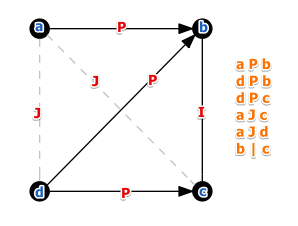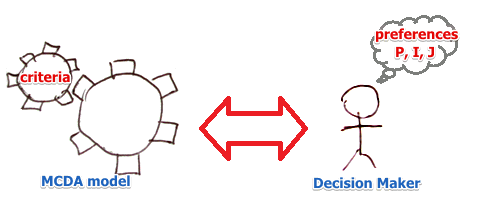ML Wiki

Modeling Preferences

To be able to find the best solution for MCDA problems we need to know subjective preferences:

So given two alternatives $a$ and $b$ a decision maker can say if

• $a \ P \ b$ or $b \ P \ a$: $a$ is preferred to $b$ - the preference relation
• $a \ I \ b$ - the indifference relation
• $a \ J \ b$ - the incomparability relation, when you cannot compare things

Main properties:

• $P$ is asymmetric
• $a \ P \ b \equiv b \ \overline{P} \ a$
• $I$ is reflexive and symmetric:
• $a \ I \ a$ and $a \ I \ b \equiv b \ I \ a$
• $J$ is irreflexible and symmetric
• $a \ \overline{J} \ a$ and $a \ J \ b \equiv b \ J \ a$

Transitivity

We can show the preferences of a decision maker with a graph:

•Preference Structures

How to build a mathematical model from statements of a decision maker?There are several preference structures that can do that:

Preferential Independence

Main Article: Preferential Independence

This is an important condition between preferences and criteria: the criteria should be preferentially independent.